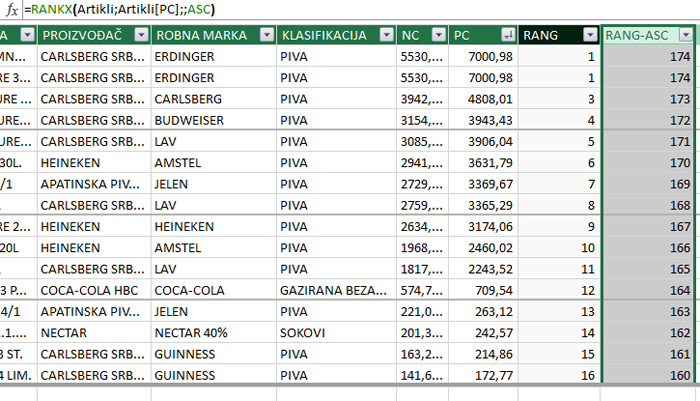# Ranking

In one of the previous “recipes” you could get acquainted with the EARLIER function. Here you could learn how to use this function to calculate the rank based on highest price of the product within a table. This can be done much more easily, by applying the RANKX function. Let’s see how it is used and what other options are also available when ranking data through DAX.

We start from the example you may have encountered in some previous posts, we work in the Artikli (Items) table which contains data on 175 products, and we analyze the column which contain sales prices (PC).

The syntax of the RANKX function is:

RANKX(<table>, <expression>[, <value>[, <order>[, <ties>]]])

This function has two mandatory parameters, the others are optional. The first is the name of the table in which we calculate the rank, and the second is the column we rank. For example, if we add a calculated column by entering the formula:

=RANKX(Artikli,Artikli[PC])and then we sort the selling prices in descending order we will see the price rank. The highest prices have a rank of 1, and the lower the prices, the lower their rank.

The following parameter represents the expression or value for which we want to calculate the rank. If e.g. we enter the price 242.57 in the formula:

=RANKX(Artikli,Artikli[PC],242.57)

it returns the value 14, which is the position of this price (rank) in relation to the others in the table.

The next parameter represents the sort direction and can be ASC (ascending order) or DESC (descending order). For example. if we enter the formula:

=RANKX(Artikli,Artikli[PC],,ASC)the ranks will be displayed in ascending order.

The last parameter tells how the ranking algorithm should behave when it finds equal values (ties). We can choose the Skip or Dense option. Skip means e.g. when we have 5 equal values ​​at the beginning, the sixth will not have rank 2 but rank 6 (5 + 1). Dense means that after the same values, the rank takes the first next. For example if the first two ranks are equal (1) the rank of the third will be the number 2.